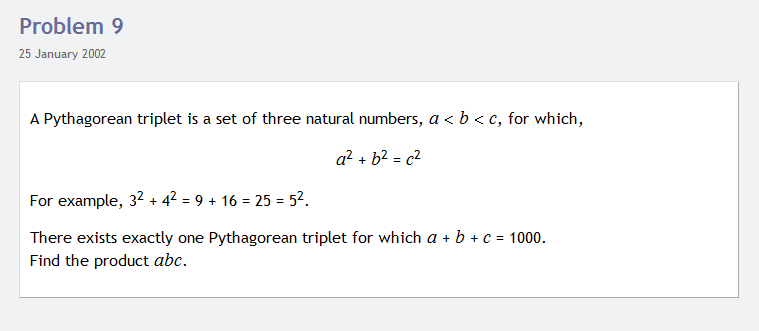Today I decided to record the process of solving a mathematical puzzle I found at the Project Euler website, in an effort to try and begin to analyze the problem solving techniques I use. My interest here is mostly in how the process unfolds, and the skills I use to solve these problems, rather than the actual problems themselves, although those are interesting. Below is the video I recorded when solving this problem.I think it would be interesting if we could create more examples of these videos in action, where we solve mathematical problems. I’m kind of inspired by Vi Hart, whose creative genius in mathematical doodling I cannot match.

Check out my discussion of a solution to the problem I solved below. Could you do this for another problem?